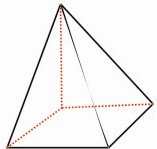HOME MATH DICTIONARY DOWNLOAD FEEDBACK DISCLAIMER
 Question: What is Pyramid ? Answer: The name comes from the Egyptian square-based pyramids. But in general a pyramid is a polyhedron formed by joining the edges of a polygon to a point to form sloping triangular faces. The volume of any pyramid is equal to 1/3 Ah, where A is the area of its base polygon and h is its height. Pyramids are generally classified by their bases. For example, the Egyptian pyramids have square bases, and are therefore called square pyramids. A triangular pyramid is also known as a tetrahedron (four sides).Example of a Pyramid: